# NCERT Solutions for Class 11 Physics chapter-10 Mechanical Properties of Fluids

## NCERT Solutions for Class 11 Physics

NCERT Solutions for class-11 Physics Chapter-10 Mechanical Properties of Fluids is prepared by our senior and renowned teachers of Physics Wallah primary focus while solving these questions of class-11 in NCERT textbook, also do read theory of this Chapter-10 Mechanical Properties of Fluids while going before solving the NCERT questions. You can download NCERT solution of all chapters from Physics Wallah in PDF.

### Chapter-10 Mechanical Properties of Fluids

Question1. Explain why
a. The blood pressure in humans is greater at the feet than at the brain
b. Atmospheric pressure at a height of about 6 km decreases to nearly half of its value at the sea level, though the height of the atmosphere is more than 100 km
c. Hydrostatic pressure is a scalar quantity even though pressure is force divided by area.

Solution :
a. The height of the blood column in the human body is more at feet than at the brain. That is why, the blood exerts more pressure at the feet than at the brain.
(Pressure = h ρ g where h = height, ρ = density of liquid and g = accleration due to gravity)
b. Density of air is the maximum near the sea level. Density of air decreases with increase in height from the surface. At a height of about 6 km, density decreases to nearly half of its value at the sea level. Atmospheric pressure is proportional to density. Hence, at a height of 6 km from the surface, it decreases to nearly half of its value at the sea level.
c. When force is applied on a liquid, the pressure in the liquid is transmitted in all directions. Hence, hydrostatic pressure does not have a fixed direction and it is a scalar physical quantity.

Question 2. Explain why
a. The angle of contact of mercury with glass is obtuse, while that of water with glass is acute.
b. Water on a clean glass surface tends to spread out while mercury on the same surface tends to form drops. (Put differently, water wets glass while mercury does not.)
c. Surface tension of a liquid is independent of the area of the surface
d. Water with detergent dissolved in it should have small angles of contact.
e. A drop of liquid under no external forces is always spherical in shape

Solution :
a. The angle between the tangent to the liquid surface at the point of contact and the surface inside the liquid is called the angle of contact (θ), as shown in the given figure.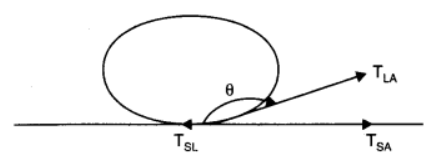Sla, Ssa, and Ssl are the respective interfacial tensions between the liquid-air, solid-air, and solid-liquid interfaces. At the line of contact, the surface forces between the three media must be in equilibrium, i.e., Cosθ = (Ssa - Sla) / Sla
The angle of contact θ , is obtuse if Ssa < Sla (as in the case of mercury on glass). This angle is acute if Ssl < Sla (as in the case of water on glass).

b. Mercury molecules (which make an obtuse angle with glass) have a strong force of attraction between themselves and a weak force of attraction toward solids. Hence, they tend to form drops.
On the other hand, water molecules make acute angles with glass. They have a weak force of attraction between themselves and a strong force of attraction toward solids. Hence, they tend to spread out.

c. Surface tension is the force acting per unit length at the interface between the plane of a liquid and any other surface. This force is independent of the area of the liquid surface. Hence, surface tension is also independent of the area of the liquid surface.

d. Water with detergent dissolved in it has small angles of contact (θ). This is because for a small θ, there is a fast capillary rise of the detergent in the cloth. The capillary rise of a liquid is directly proportional to the cosine of the angle of contact (θ). If θ is small, then cosθ will be large and the rise of the detergent water in the cloth will be fast.

e. A liquid tends to acquire the minimum surface area because of the presence of surface tension. The surface area of a sphere is the minimum for a given volume. Hence, under no external forces, liquid drops always take spherical shape.

Question 3. Fill in the blanks using the word(s) from the list appended with each statement:
a. With lowering of temperature surface tension of liquids generally __________ (decrease/increase)
b. Shearing force is proportional to ______ for solids having elastic modulus of rigidity, while it is proportional to __________ for fluids. (Rate of shear strain/shear strain)
c. Viscosity of _______ increases with the temperature while for _____ it decreases. (Liquids/gases)
d. For model of an aircraft inside a wind tunnel, turbulence occurs at a speed ______   than the speed for turbulence for an actual plane. (Lesser / greater)
e. A fluid in steady flow experiences increases in its flow speed at constrictions as according to ____ and the decrease of pressure there comes from ___________. (Bernoulli’s principle /conservation of mass )

Solution :
a. Decreases
b. Shear strain, rate of shear strain
c. Gases, liquid
d. Greater
e. Conservation of mass, Bernoulli’s principle

Question 4. Explain why
a. To keep a piece of paper horizontal, you should blow over, not under, it
b. When we try to close a water tap with our fingers, fast jets of water gush through the openings between our fingers
c. The size of the needle of a syringe controls flow rate better than the thumb pressure exerted by a doctor while administering an injection
d. A fluid flowing out of a small hole in a vessel results in a backward thrust on the vessel
e. A spinning cricket ball in air does not follow a parabolic trajectory.

Solution :
a.  When we blow over the paper, the velocity of air blow increases and hence pressure of air on it decreases (according to Beroulli's Theorem), where as pressure of air blow the paper is atmospheric. Hence, the paper stays horizontal.
b. By ddoing so the area of outlet of water jet is reduced, so velocity of water increases according to equation of continuity, Area × Velocity = Constant.
c. The small opening of a syringe needle controls the velocity of the blood flowing out. This is because of the equation of continuity. At the constriction point of the syringe system, the flow rate suddenly increases to a high value for a constant thumb pressure applied.
d. When a fluid flows out from a small hole in a vessel, the vessel receives a backward thrust. A fluid flowing out from a small hole has a large velocity according to the equation of continuity:
Area × Velocity = Constant
According to the law of conservation of momentum, the vessel attains a backward velocity because there are no external forces acting on the system.
e. A spinning cricket ball has two simultaneous motions – rotatory and linear. These two types of motion oppose the effect of each other. This decreases the velocity of air flowing below the ball. Hence, the pressure on the upper side of the ball becomes lesser than that on the lower side. An upward force acts upon the ball. Therefore, the ball takes a curved path. It does not follow a parabolic path.

Question 5. A 50 kg girl wearing high heel shoes balances on a single heel. The heel is circular with a diameter 1.0 cm. What is the pressure exerted by the heel on the horizontal floor?

Solution :
Mass of the girl, m = 50 kg
Diameter of the heel, d = 1 cm = 0.01 m
Radius of the heel, r = d/2 = 0.005 m
Area of the heel = πr2
= π (0.005)2
= 7.85 × 10–5 m2
Force exerted by the heel on the floor:
F = mg
= 50 × 9.8
= 490 N
Pressure exerted by the heel on the floor:
P = Force / Area
= 490 / (7.85 × 10-5)  =  6.24 × 106 Nm-2
Therefore, the pressure exerted by the heel on the horizontal floor is

24 × 106 Nm–2.

Question 6. Toricelli’s barometer used mercury. Pascal duplicated it using French wine of density 984 kg m–3. Determine the height of the wine column for normal atmospheric pressure.

Solution :
Density of mercury, ρ1 = 13.6 × 103 kg/m3 Height of the mercury column, h1 = 0.76 m
Density of French wine, ρ2 = 984 kg/m3
Height of the French wine column = h2
Acceleration due to gravity, g = 9.8 m/s2
The pressure in both the columns is equal, i.e.,
Pressure in the mercury column = Pressure in the French wine column
ρ1h1g  =  ρ2h2g
h2 = ρ1h1 / ρ2
= 13.6 × 103 × 0.76 / 984  =  10.5 m
Hence, the height of the French wine column for normal atmospheric pressure is 10.5 m.

Question 7. A vertical off-shore structure is built to withstand a maximum stress of 109 Pa. Is the structure suitable for putting up on top of an oil well in the ocean? Take the depth of the ocean to be roughly 3 km, and ignore ocean currents.

Solution :
The maximum allowable stress for the structure, P = 109 Pa
Depth of the ocean, d = 3 km = 3 × 103 m
Density of water, ρ = 103 kg/m3
Acceleration due to gravity, g = 9.8 m/s2
The pressure exerted because of the sea water at depth, d = ρdg
= 3 × 103 × 103 × 9.8
= 2.94 × 107 Pa
The maximum allowable stress for the structure (109 Pa) is greater than the pressure of the sea water (2.94 × 107 Pa). The pressure exerted by the ocean is less than the pressure that the structure can withstand. Hence, the structure is suitable for putting up on top of an oil well in the ocean.

Question 8. A hydraulic automobile lift is designed to lift cars with a maximum mass of 3000 kg. The area of cross-section of the piston carrying the load is 425 cm2. What maximum pressure would the smaller piston have to bear?

Solution :
The maximum mass of a car that can be lifted, m = 3000 kg
Area of cross-section of the load-carrying piston, A = 425 cm= 425 × 10–4 m2
The maximum force exerted by the load, F = mg
= 3000 × 9.8
= 29400 N
The maximum pressure exerted on the load-carrying piston, P = F/A
29400 / (425 × 10-4)
= 6.917 × 105 Pa
Pressure is transmitted equally in all directions in a liquid. Therefore, the maximum pressure that the smaller piston would have to bear is 6.917 × 105 Pa.

Question 9. A U-tube contains water and methylated spirit separated by mercury. The mercury columns in the two arms are in level with 10.0 cm of water in one arm and 12.5 cm of spirit in the other. What is the specific gravity of spirit?

Solution :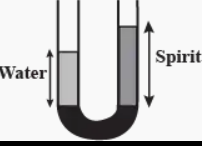Height of the spirit column, h1 = 12.5 cm = 0.125 m
Height of the water column, h2 = 10 cm = 0.1 m
P0 = Atmospheric pressure
ρ1 = Density of spirit
ρ2 = Density of water
Pressure at point B = P0 + ρ1h1g
Pressure at point D = P0 + ρ2h2g
Pressure at points B and D is the same.
P0 + ρ1h1g  =  P0 + ρ2h2g
ρ1 / ρ2  =  h2 / h1
= 10 / 12.5  =  0.8
Therefore, the specific gravity of spirit is 0.8.

Question10. In problem 10.9, if 15.0 cm of water and spirit each are further poured into the respective arms of the tube, what is the difference in the levels of mercury in the two arms? (Specific gravity of mercury = 13.6).

Solution :
Height of the water column, h1 = 10 + 15 = 25 cm
Height of the spirit column, h2 = 12.5 + 15 = 27.5 cm
Density of water, ρ1 = 1 g cm–3
Density of spirit, ρ2 = 0.8 g cm–3
Density of mercury = 13.6 g cm–3
Let h be the difference between the levels of mercury in the two arms.
Pressure exerted by height h, of the mercury column:
= hρg
= h × 13.6g … (i)
Difference between the pressures exerted by water and spirit:
= ρ1h1g - ρ2h2g
= g(25 × 1 – 27.5 × 0.8)
= 3g … (ii)
Equating equations (i) and (ii), we get:
13.6 hg = 3g
h = 0.220588 ≈ 0.221 cm
Hence, the difference between the levels of mercury in the two arms is 0.221 cm.

Question11. Can Bernoulli’s equation be used to describe the flow of water through a rapid in a river? Explain.

Solution :
Bernoulli’s equation cannot be used to describe the flow of water through a rapid in a river because of the turbulent flow of water. This principle can only be applied to a streamline flow.

Question 12. Does it matter if one uses gauge instead of absolute pressures in applying Bernoulli’s equation? Explain.

Solution :
No, it does not matter if one uses gauge pressure instead of absolute pressure while applying Bernoulli’s equation. The two points where Bernoulli’s equation is applied should have significantly different atmospheric pressures.

Question 13. Glycerine flows steadily through a horizontal tube of length 1.5 m and radius 1.0 cm. If the amount of glycerine collected per second at one end is 4.0 × 10–3 kg s–1, what is the pressure difference between the two ends of the tube? (Density of glycerine = 1.3 × 103 kg m–3 and viscosity of glycerine = 0.83 Pa s). [You may also like to check if the assumption of laminar flow in the tube is correct].

Solution :
Length of the horizontal tube, l = 1.5 m
Radius of the tube, r = 1 cm = 0.01 m
Diameter of the tube, d = 2r = 0.02 m
Glycerine is flowing at a rate of 4.0 × 10–3 kg s–1.
M = 4.0 × 10–3 kg s–1
Density of glycerine, ρ = 1.3 × 103 kg m–3
Viscosity of glycerine, η = 0.83 Pa s
Volume of glycerine flowing per sec:
V = M / ρ
= 4 × 10-3 / (1.3 × 103)  =  3.08 × 10-6 m3s-1
According to Poiseville’s formula, we have the relation for the rate of flow:
V = πpr4 / 8ηl
Where, p is the pressure difference between the two ends of the tube
∴ p = V8ηl / πr4
= 3.08 × 10-6 × 8 × 0.83 × 1.5 / [ π × (0.01)4 ]
= 9.8 × 102 Pa
Reynolds’ number is given by the relation:
R = 4pV / πdη
= 4 × 1.3 × 103 × 3.08 × 10-6 / ( π × 0.02 × 0.83)
= 0.3
Reynolds’ number is about 0.3. Hence, the flow is laminar.

Question 14. In a test experiment on a model aeroplane in a wind tunnel, the flow speeds on the upper and lower surfaces of the wing are 70 m s–1and 63 m s–1 respectively. What is the lift on the wing if its area is 2.5 m2? Take the density of air to be 1.3 kg m–3.

Solution :
Speed of wind on the upper surface of the wing, V1 = 70 m/s
Speed of wind on the lower surface of the wing, V2 = 63 m/s
Area of the wing, A = 2.5 m2
Density of air, ρ = 1.3 kg m–3
According to Bernoulli’s theorem, we have the relation:
P+ (1/2)ρV12 = P+ (1/2)ρV22
P2 - P1 = (1/2) ρ (V12 - V22)
Where,
P1 = Pressure on the upper surface of the wing
P2 = Pressure on the lower surface of the wing
The pressure difference between the upper and lower surfaces of the wing provides lift to the aeroplane.
Lift on the wing = (P2 - P1) A
= (1/2) ρ (V12 - V22) A
= (1/2) × 1.3 × [ 702 - 632 ] × 2.5
= 1512.87 N  =  1.51 × 103 N
Therefore, the lift on the wing of the aeroplane is 1.51 × 103 N.

Question 15. Figures 10.23 (a) and (b) refer to the steady flow of a (non-viscous) liquid. Which of the two figures is incorrect? Why?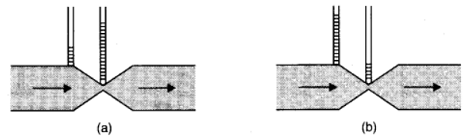Solution :

Fig. (a) is incorrect. Accoridng to equation of continuity,

A1V1 = A2V2

i.e., av = Constant, where area of cross-section of tube is less, the velcoity of liquid flow is more.

So the velocity of liquid flow at a constriction of tube is more than the other portion of tube.

Accroding to Bernoulli's Theorem, P + 1/2ρv= Constant,

where v is more, P is less and vice versa.

Question 16. The cylindrical tube of a spray pump has a cross-section of 8.0 cm2 one end of which has 40 fine holes each of diameter 1.0 mm. If the liquid flow inside the tube is 1.5 m min–1, what is the speed of ejection of the liquid through the holes?

Solution :
Area of cross-section of the spray pump, A1 = 8 cm= 8 × 10–4 m2
Number of holes, n = 40
Diameter of each hole, d = 1 mm = 1 × 10–3 m
Radius of each hole, r = d/2 = 0.5 × 10–3 m
Area of cross-section of each hole, a = πr= π (0.5 × 10–3)2 m2
Total area of 40 holes, A= n × a
= 40 × π (0.5 × 10–3)2 m2
= 31.41 × 10–6 m2
Speed of flow of liquid inside the tube, V= 1.5 m/min = 0.025 m/s
Speed of ejection of liquid through the holes = V2
According to the law of continuity, we have:
A1V1 = A2V2
V2 = A1V1 / A2
= 8 × 10-4 × 0.025 / (31.61 × 10-6)
= 0.633 m/s
Therefore, the speed of ejection of the liquid through the holes is 0.633 m/s.

Question 17. A U-shaped wire is dipped in a soap solution, and removed. The thin soap film formed between the wire and the light slider supports a weight of 1.5 × 10–2 N (which includes the small weight of the slider). The length of the slider is 30 cm. What is the surface tension of the film?

Solution :
The weight that the soap film supports, W = 1.5 × 10–2 N
Length of the slider, l = 30 cm = 0.3 m
A soap film has two free surfaces.
∴ Total length = 2l = 2 × 0.3 = 0.6 m
Surface tension, S = Force or Weight / 2l
= 1.5 × 10-2 / 0.6
= 2.5 × 10-2 N/m
Therefore, the surface tension of the film is 2.5 × 10–2 N m–1.

Question 18. Figure 10.24 (a) shows a thin liquid film supporting a small weight = 4.5 × 10–2 N. What is the weight supported by a film of the same liquid at the same temperature in Fig. (b) and (c)? Explain your answer physically.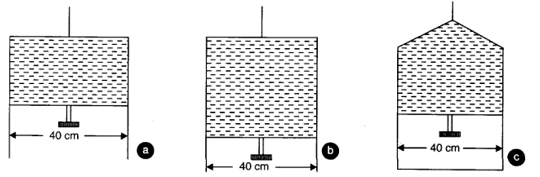Solution :

Take case (a): The length of the liquid film supported by the weight, l = 40 cm = 0.4 cm
The weight supported by the film, W = 4.5 × 10–2 N
A liquid film has two free surfaces.
∴ Surface tension = W / 2l
= 4.5 × 10-2 / (2 × 0.4)  = 5.625 × 10-2 N/m
In all the three figures, the liquid is the same. Temperature is also the same for each case. Hence, the surface tension in figure (b) and figure (c) is the same as in figure (a), i.e., 5.625 × 10–2 N m–1.
Since the length of the film in all the cases is 40 cm, the weight supported in each case is 4.5 × 10–2 N.

Question19. What is the pressure inside the drop of mercury of radius 3.00 mm at room temperature? Surface tension of mercury at that temperature (20°C) is 4.65 × 10–1 N m–1. The atmospheric pressure is 1.01 × 105 Pa. Also give the excess pressure inside the drop.

Solution :
Radius of the mercury drop, r = 3.00 mm = 3 × 10–3 m
Surface tension of mercury, S = 4.65 × 10–1 N m–1
Atmospheric pressure, P0 = 1.01 × 105 Pa
Total pressure inside the mercury drop
= Excess pressure inside mercury + Atmospheric pressure
= 2S / r + P0
= [ 2 × 4.65 × 10-1 / (3 × 10-3) ] + 1.01 × 105
= 1.0131 × 105
= 1.01 × 105 Pa
Excess pressure = 2S / r
= [ 2 × 4.65 × 10-1 / (3 × 10-3) ] = 310 Pa.

Question20. What is the excess pressure inside a bubble of soap solution of radius 5.00 mm, given that the surface tension of soap solution at the temperature (20 °C) is 2.50 × 10–2 N m–1? If an air bubble of the same dimension were formed at depth of 40.0 cm inside a container containing the soap solution (of relative density 1.20), what would be the pressure inside the bubble? (1 atmospheric pressure is 1.01 × 105 Pa).

Solution :
Excess pressure inside the soap bubble is 20 Pa;
Pressure inside the air bubble is 1.06 × 105 Pa
Soap bubble is of radius, r = 5.00 mm = 5 × 10–3 m
Surface tension of the soap solution, S = 2.50 × 10–2 Nm–1
Relative density of the soap solution = 1.20
∴ Density of the soap solution, ρ = 1.2 × 103 kg/m3
Air bubble formed at a depth, h = 40 cm = 0.4 m
Radius of the air bubble, r = 5 mm = 5 × 10–3 m
1 atmospheric pressure = 1.01 × 105 Pa
Acceleration due to gravity, g = 9.8 m/s2
Hence, the excess pressure inside the soap bubble is given by the relation:
P = 4S / r
= 4 × 2.5 × 10-2 / 5 × 10-3
= 20 Pa
Therefore, the excess pressure inside the soap bubble is 20 Pa.
The excess pressure inside the air bubble is given by the relation:
P' = 2S / r
= 2 × 2.5 × 10-2 / (5 × 10-3)
= 10 Pa
Therefore, the excess pressure inside the air bubble is 10 Pa.
At a depth of 0.4 m, the total pressure inside the air bubble
= Atmospheric pressure + hρg + P’
= 1.01 × 105 + 0.4 × 1.2 × 103 × 9.8 + 10
= 1.06 × 105 Pa
Therefore, the pressure inside the air bubble is 1.06 × 105 Pa.

Question21. A tank with a square base of area 1.0 m2 is divided by a vertical partition in the middle. The bottom of the partition has a small-hinged door of area 20 cm2. The tank is filled with water in one compartment, and an acid (of relative density 1.7) in the other, both to a height of 4.0 m. compute the force necessary to keep the door close.

Solution :
Base area of the given tank, A = 1.0 m2
Area of the hinged door, a = 20 cm= 20 × 10–4 m2
Density of water, ρ1 = 103 kg/m3
Density of acid, ρ2 = 1.7 × 103 kg/m3
Height of the water column, h1 = 4 m
Height of the acid column, h2 = 4 m
Acceleration due to gravity, g = 9.8
Pressure due to water is given as:
P1 = h1ρ1g
= 4 × 103 × 9.8  =  3.92 × 104 Pa
Pressure due to acid is given as:
P2 = h2ρ2g
= 4 × 1.7 × 103 × 9.8  =  6.664 × 104 Pa
Pressure difference between the water and acid columns:
ΔP = P2 - P1
= 6.664 × 104 - 3.92 × 104
= 2.744 × 104 Pa
Hence, the force exerted on the door = ΔP × a
= 2.744 × 104 × 20 × 10–4
= 54.88 N
Therefore, the force necessary to keep the door closed is 54.88 N.

Question22. A manometer reads the pressure of a gas in an enclosure as shown in Fig. 10.25 (a) When a pump removes some of the gas, the manometer reads as in Fig. 10.25 (b) The liquid used in the manometers is mercury and the atmospheric pressure is 76 cm of mercury.
a. Give the absolute and gauge pressure of the gas in the enclosure for cases (a) and (b), in units of cm of mercury.
b. How would the levels change in case (b) if 13.6 cm of water (immiscible with mercury) are poured into the right limb of the manometer? (Ignore the small change in the volume of the gas).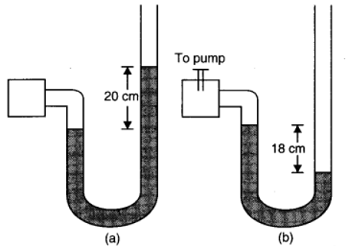Solution :
a. For figure (a)
Atmospheric pressure, P= 76 cm of Hg
Difference between the levels of mercury in the two limbs gives gauge pressure
Hence, gauge pressure is 20 cm of Hg.
Absolute pressure = Atmospheric pressure + Gauge pressure
= 76 + 20 = 96 cm of Hg

For figure (b)
Difference between the levels of mercury in the two limbs = –18 cm
Hence, gauge pressure is –18 cm of Hg.
Absolute pressure = Atmospheric pressure + Gauge pressure
= 76 cm – 18 cm = 58 cm

b. 13.6 cm of water is poured into the right limb of figure (b).
Relative density of mercury = 13.6
Hence, a column of 13.6 cm of water is equivalent to 1 cm of mercury.
Let h be the difference between the levels of mercury in the two limbs.
The pressure in the right limb is given as:
PR = Atmospheric pressure + 1 cm of Hg
= 76 + 1 = 77 cm of Hg … (i)
The mercury column will rise in the left limb.
Hence, pressure in the left limb, PL = 58 + h               .....(ii)
Equating equations (i) and (ii), we get:
77 = 58 + h
∴h = 19 cm
Hence, the difference between the levels of mercury in the two limbs will be 19 cm.

Question23. Two vessels have the same base area but different shapes. The first vessel takes twice the volume of water that the second vessel requires to fill upto a particular common height. Is the force exerted by the water on the base of the vessel the same in the two cases? If so, why do the vessels filled with water to that same height give different readings on a weighing scale?

Solution :
Two vessels having the same base area have identical force and equal pressure acting on their common base area. Since the shapes of the two vessels are different, the force exerted on the sides of the vessels has non-zero vertical components. When these vertical components are added, the total force on one vessel comes out to be greater than that on the other vessel. Hence, when these vessels are filled with water to the same height, they give different readings on a weighing scale.

Question24. During blood transfusion the needle is inserted in a vein where the gauge pressure is 2000 Pa. At what height must the blood container be placed so that blood may just enter the vein? [Use the density of whole blood from Table 10.1].

Solution :
Gauge pressure, P = 2000 Pa
Density of whole blood, ρ = 1.06 × 10kg m–3
Acceleration due to gravity, g = 9.8 m/s2
Height of the blood container = h
Pressure of the blood container, P = hρg
∴ h = P / ρg
= 2000 / (1.06 × 103 × 9.8)
= 0.1925 m
The blood may enter the vein if the blood container is kept at a height greater than 0.1925 m, i.e., about 0.2 m.

Question25. In deriving Bernoulli’s equation, we equated the work done on the fluid in the tube to its change in the potential and kinetic energy. (a) What is the largest average velocity of blood flow in an artery of diameter 2 × 10–3 m if the flow must remain laminar? (b) Do the dissipative forces become more important as the fluid velocity increases? Discuss qualitatively.

Solution :
(a) If dissipative forces are present, hen some forces in liquid flow due to pressure difference is spent against dissipative forces. Due to which the pressure drop becomes large.
(b) The dissipative forces become more important with increasing flow velocity, because of turbulence.

Question26. (a) What is the largest average velocity of blood flow in an artery of radius 2 × 10–3 m if the flow must remain laminar? (b) What is the corresponding flow rate? (Take viscosity of blood to be 2.084 × 10–3 Pa s).

Solution :
(a) Radius of the artery, r = 2 × 10–3 m
Diameter of the artery, d = 2 × 2 × 10–3 m = 4 × 10– 3 m
Viscosity of blood, η = 2.084 X 10-3 Pa s
Density of blood, ρ = 1.06 × 103 kg/m3
Reynolds’ number for laminar flow, NR = 2000
The largest average velocity of blood is given by the relation:
Varg = NRη / ρd
= 2000 × 2.084 × 10-3 / (1.06 × 103 × 4 × 10-3)
= 0.983 m/s
Therefore, the largest average velocity of blood is 0.983 m/s.

(b) Flow rate is given by the relation:
R = π r2Vavg
= 3.14 × (2 × 10-3)2 × 0.983
= 1.235 × 10-5 m3s-1
Therefore, the corresponding flow rate is 1.235 × 10-5 m3s-1.

Question27. A plane is in level flight at constant speed and each of its two wings has an area of 25 m2. If the speed of the air is 180 km/h over the lower wing and 234 km/h over the upper wing surface, determine the plane’s mass. (Take air density to be 1 kg m–3).

Solution :
The area of the wings of the plane, A = 2 × 25 = 50 m2
Speed of air over the lower wing, V1 = 180 km/h = 50 m/s
Speed of air over the upper wing, V2 = 234 km/h = 65 m/s
Density of air, ρ = 1 kg m–3
Pressure of air over the lower wing = P1
Pressure of air over the upper wing= P2
The upward force on the plane can be obtained using Bernoulli’s equation as:
P1 + (1/2) ρ V12  =  P2 + (1/2) ρ V22
P1 - P2 = (1/2) ρ ( V22 - V12)   .....(i)
The upward force (F) on the plane can be calculated as:
(P1 - P2)A  =  (1/2) ρ ( V22 - V12) A
= (1/2) × 1 × (652 - 502) × 50
= 43125 N
Using Newton’s force equation, we can obtain the mass (m) of the plane as:
F = mg
∴ m = 43125 / 9.8 = 4400.51 kg
∼ 4400 kg
Hence, the mass of the plane is about 4400 kg.

Question28. In Millikan’s oil drop experiment, what is the terminal speed of an uncharged drop of radius 2.0 × 10–5 m and density 1.2 × 103 kg m–3? Take the viscosity of air at the temperature of the experiment to be 1.8 × 10–5 Pa s. How much is the viscous force on the drop at that speed? Neglect buoyancy of the drop due to air.

Solution :
Terminal speed = 5.8 cm/s
Viscous force = 3.9 × 10–10 N
Radius of the given uncharged drop, r = 2.0 × 10–5 m
Density of the uncharged drop, ρ = 1.2 × 103 kg m–3
Viscosity of air, η = 1.8 × 10-5 Pa s
Density of air (ρ0) can be taken as zero in order to neglect buoyancy of air.
Acceleration due to gravity, g = 9.8 m/s2
Terminal velocity (v) is given by the relation:
v = 2r2 × (ρ - ρ0) g / 9η
= 2 × (2 × 10-5)2 (1.2 × 103 - 0 ) × 9.8 / (9 × 1.8 × 10-5)
= 5.8 × 10-2 m/s
= 5.8 cm s-1
Hence, the terminal speed of the drop is 5.8 cm s–1.
The viscous force on the drop is given by:
F = 6πηrv
∴ F = 6 × 3.14 × 1.8 × 10-5 × 2 × 10-5 × 5.8 × 10-2
= 3.9 × 10-10 N
Hence, the viscous force on the drop is 3.9 × 10–10 N.

Question 29. Mercury has an angle of contact equal to 140° with soda lime glass. A narrow tube of radius 1.00 mm made of this glass is dipped in a trough containing mercury. By what amount does the mercury dip down in the tube relative to the liquid surface outside? Surface tension of mercury at the temperature of the experiment is 0.465 N m–1. Density of mercury = 13.6 × 103 kg m–3.

Solution :
Angle of contact between mercury and soda lime glass, θ = 140°
Radius of the narrow tube, r = 1 mm = 1 × 10–3 m
Surface tension of mercury at the given temperature, s = 0.465 N m–1
Density of mercury, ρ =13.6 × 103 kg/m3
Dip in the height of mercury = h
Acceleration due to gravity, g = 9.8 m/s2
Surface tension is related with the angle of contact and the dip in the height as:
s = hρgr / 2 Cosθ
∴ h = 2s Cosθ / ρgr
= 2 × 0.465  × Cos1400 / (1  × 10-3  × 13.6  × 103 × 9.8)
= -0.00534 m
= -5.34 m
Here, the negative sign shows the decreasing level of mercury. Hence, the mercury level dips by 5.34 mm.

Question30. Two narrow bores of diameters 3.0 mm and 6.0 mm are joined together to form a U-tube open at both ends. If the U-tube contains water, what is the difference in its levels in the two limbs of the tube? Surface tension of water at the temperature of the experiment is 7.3 × 10–2 N m–1. Take the angle of contact to be zero and density of water to be 1.0 × 103 kg m–3 (g = 9.8 m s–2).

Solution :
Diameter of the first bore, d1 = 3.0 mm = 3 × 10–3 m
Hence, the radius of the first bore, r1 = d1/ 2  =  1.5 × 10-3 m
Diameter of the first bore, d2 = 6.0 mm = 6 × 10–3 mm
Hence, the radius of the first bore, r2 = d2/ 2  =  3 × 10-3 m
Surface tension of water, s = 7.3 × 10–2 N m–1
Angle of contact between the bore surface and water, θ= 0
Density of water, ρ =1.0 × 103 kg/m–3
Acceleration due to gravity, g = 9.8 m/s2
Let h1 and hbe the heights to which water rises in the first and second tubes respectively. These heights are given by the relations:
h1 = 2s Cosθ / r1ρg      .....(i)
h2 = 2s Cosθ / r2ρg      .....(ii)
The difference between the levels of water in the two limbs of the tube can be calculated as: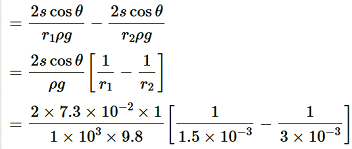= 4.966 × 10-3 m

= 4.97 mm

Hence, the difference between levels of water in the two bores is 4.97 mm.

Question31. (a) It is known that density ρ of air decreases with height y as ρ0e-y/y0 Where ρ0 = 1.25 kg m-3
is the density at sea level, and y0 is a constant. This density variation is called the law of atmospheres. Obtain this law assuming that the temperature of atmosphere remains a constant (isothermal conditions). Also assume that the value of gremains constant.
(b) A large He balloon of volume 1425 m3 is used to lift a payload of 400 kg. Assume that the balloon maintains constant radius as it rises. How high does it rise?
[Take y0= 8000 m and ρHe = 0.18 kg m-3 ]

Solution :
(a) Volume of the balloon, V = 1425 m3
Mass of the payload, m = 400 kg
Acceleration due to gravity, g = 9.8 m/s2
y0 = 8000 m
ρHe = 0.18 kg m-3
ρ0 = 1.25 kg m-3
Density of the balloon = ρ
Height to which the balloon rises = y
Density (ρ) of air decreases with height (y) as:
ρ = ρ0e-y/y0
ρ / ρ= e-y/y0     ....(i)

This density variation is called the law of atmospherics.
It can be inferred from equation (i) that the rate of decrease of density with height is directly proportional to ρ, i.e.,
-(dρ / dy) ∝ ρ
(dρ / dy) = -kρ
(dρ / ρ) = -k dy
Where, k is the constant of proportionality
Height changes from 0 to y, while density changes from ρ0 to ρ
Integrating the sides between these limits, we get: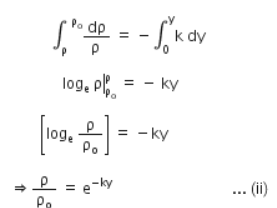Comparing equations (i) and (ii), we get: y0 = 1/k
k = 1/y0   ....(iii)
From equations (i) and (iii), we get
ρ = ρ0e-y/y0
(b) Density ρ = Mass / Volume
= (Mass of the payload + Mass of helium) / Volume
( m + VρHe) / V
= (400 + 1425 × 0.18) / 1425
= 0.46 kg m-3
From equations (ii) and (iii), we can obtain y as:
ρ = ρ0e-y/y0
loge(ρ / ρ0)  =  -y / y0
∴ y = - 8000 × loge(0.46 / 1.25)
= -8000 × (-1)
= 8000 m  =  8 km
Hence, the balloon will rise to a height of 8 km.